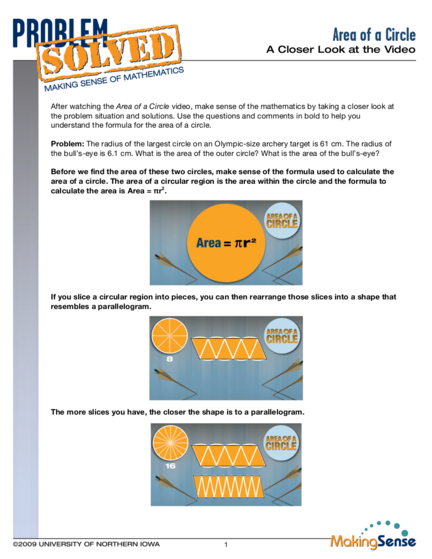# Word Problems of CircleContributed by:This pdf contains word problems on circumference and area of circles.
1. Area of a Circle
A Closer Look at the Video
After watching the Area of a Circle video, make sense of the mathematics by taking a closer look at
understand the formula for the area of a circle.
Problem: The radius of the largest circle on an Olympic-size archery target is 61 cm. The radius of
the bull’s-eye is 6.1 cm. What is the area of the outer circle? What is the area of the bull’s-eye?
Before we find the area of these two circles, make sense of the formula used to calculate the
area of a circle. The area of a circular region is the area within the circle and the formula to
calculate the area is Area = πr2.
If you slice a circular region into pieces, you can then rearrange those slices into a shape that
resembles a parallelogram.
The more slices you have, the closer the shape is to a parallelogram.
1
2. Area of a Circle
A Closer Look at the Video
If you had an infinite number of slices, then the circle would form a parallelogram.
How do you find the area of a parallelogram? The formula used to calculate area of a
parallelogram is base times height (Area of a parallelogram = bh).
What is the height of the parallelogram shown above? What is the base of the parallelogram
shown above? The height of the parallelogram is equal to the radius of the circle. The base of the
parallelogram is one half of the circumference of the circle.
What is one half of the circumference of a circle? The circumference of any circle is π times the
diameter (πd) and the diameter of a circle is twice the length of the radius (d = 2r). Therefore, the
circumference of any circle is 2πr. The base of the parallelogram is one half of the circumference of
the circle, so the base = ½(2πr) or base = πr.
2
3. Area of a Circle
A Closer Look at the Video
Find the area of the parallelogram shown below. The area of a parallelogram is base times height
(A = bh). Since the height of this parallelogram is r and the base is πr, the area is (πr)r or πr2.
Since the area of the parallelogram is equal to the area of a circle, the area of the circle is
equal to (πr)r, or πr2.
Area of this
parallelogram = πr2
Area of a circle = πr2
Use this formula to find the area of the outer circle and the area of the bull’s-eye of the archery
target. The area of a circle is π times the radius squared. The diameter of the outer circle on an
Olympic-size archery target is 122 cm and the radius is 61 cm. The diameter of the bull’s-eye is 12.2
cm and the radius is 6.1 cm.
Area of the outer circle Area of the bull’s-eye
A= π r 2
A = π r2
= π · 612 sq. cm = π · 6.12 sq. cm
= π · 3721 sq. cm = π · 37.21 sq. cm
= 3721π sq. cm = 37.21π sq. cm
Substitute 3.14 for π Substitute 3.14 for π
≈ 3721 3.14 ≈ 37.21 3.14
≈ 11683.94 sq. cm ≈ 116.8394 sq. cm
The area of the outer circle is The area of the bull’s-eye is
You can report the area of the outer circle as 3721π sq. cm and the bull’s-eye as 37.21π sq. cm, or
you can substitute 3.14 for pi and report the areas as approximately 11,684 sq. cm and 116.8 sq.
cm. Since the radius of the outer circle was measured to the nearest centimeter, it is more accurate
to report the area of the circle to the nearest square centimeter. Likewise, since the radius of the
bull’s-eye was measured to the nearest one-tenth centimeter it is more accurate to report the area of
the bull’s-eye to the nearest one-tenth square centimeter.
3
4. Area of a Circle
A Closer Look at the Video
Compare the radius of the outer circle and the radius of the bull’s-eye. Compare the area of
the outer circle and the area of the bull’s-eye. What do you notice? The area of the outer circle is
100 times larger than the area of the bull’s-eye even though the radius of the outer circle is only 10
times the length of the radius of the bull’s-eye.
x 10
x 100
If you would like to further investigate this relationship, watch the Changing Dimensions: Area video.
For more information on pi and the area of any circle, watch the What is π? video.
4